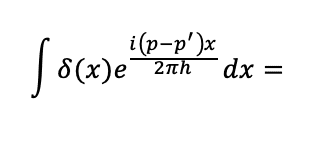# Integral Involving the Dirac Delta Function

Homework Statement:
What is the integral of the following equation?
Relevant Equations:
See below for the equation.## Answers and Replies

What are your limits of integral?

What are your limits of integral?
-∞ to +∞

-∞ to +∞
So what do you think?

So what do you think?
1?

1?
Yes

Yes
I know intuitively that the integral is 1, can you explain it to me mathematically?

PeroK
Science Advisor
Homework Helper
Gold Member
2020 Award
I know intuitively that the integral is 1, can you explain it to me mathematically?
By definition:
$$\int_{-\infty}^{+\infty}\delta(x) f(x) dx = f(0)$$

•PhDeezNutz and gabriellelee
wrobel
Science Advisor
Actually it is not an integral it just a symbol. As well as ##\delta-##function is not a function in usual sense. These things are clarified in functional analysis

vanhees71
Science Advisor
Gold Member
Hm, is this an exercise in a physics course or a trick question in a mathematics course? In the latter case, I think, the answer is that the integral is undefined, because the exponential function is not a proper test function in the domain (of, e.g., Schwartz functions), the Dirac distribution is defined on.

wrobel
Science Advisor
There is no sense to speak about integrals here. It is nothing more than symbol in this context.
A space of test functions is a conditional question. In different problems this space is introduced differently this depends of problem we consider . Loosely speaking, the smaller space of test functions the bigger space of generalized functions.

For example, consider a subspace of ##\mathcal D'(\mathbb{R})## that consists of generalized functions with compact support. Such generalized functions are naturally defined on ##C^\infty(\mathbb{R})##.

Last edited:
•jasonRF
vanhees71
Science Advisor
Gold Member
I'm only a bit familiar with the formalism, but I don't think that ##\exp(\mathrm{i} x)## is a proper test function in any case. I'm a bit unsure, whether the Dirac ##\delta## function (which has compact support, namely only 1 point) is defined on the entire ##C^{\infty}(\mathbb{R})##. Isn't it usually either ##C_0^{\infty}(\mathbb{R})## or the "Schwartz space of quickly falling functions"?

wrobel
Science Advisor
What is the problem to define the Dirac δ function on ##C^\infty(\mathbb{R})##?
Just put
$$(\delta, \varphi):=\varphi(0),\quad \varphi\in C^\infty(\mathbb{R})$$
This is a continuous linear functional with respect the standard topology in ##C^\infty(\mathbb{R})##

vanhees71
Science Advisor
Gold Member
I don't know. Maybe you can do that, but in quantum mechanics you often have confused students, because they think ##\exp(\mathrm{i} p x)## is a "eigenstate of the momentum operator", but it is not square integrable nor in the domain of the momentum operator as an essentially self-adjoint operator. Here you need the rigged-Hilbert space description!